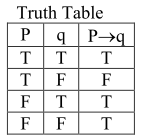# Consider the following three statements :`
Question:

Consider the following three statements :

(A) If $3+3=7$ then $4+3=8$.

(B) If $5+3=8$ then earth is flat.

(C) If both (A) and (B) are true then $5+6=17$.

Then, which of the following statements is correct ?

1. (A) is false, but (B) and (C) are true

2. (A) and (C) are true while (B) is false

3. (A) is true while (B) and (C) are false

4. (A) and (B) are false while (C) is true

Correct Option: , 2

Solution: